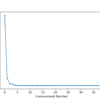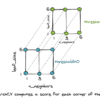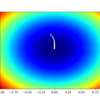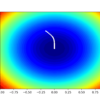# Archive | Optimization## Optimization for Machine Learning Crash Course

Optimization for Machine Learning Crash Course. Find function optima with Python in 7 days. All machine learning models involve optimization. As a practitioner, we optimize for the most suitable hyperparameters or the subset of features. Decision tree algorithm optimize for the split. Neural network optimize for the weight. Most likely, we use computational algorithms to […]## A Gentle Introduction to Particle Swarm Optimization

Particle swarm optimization (PSO) is one of the bio-inspired algorithms and it is a simple one to search for an optimal solution in the solution space. It is different from other optimization algorithms in such a way that only the objective function is needed and it is not dependent on the gradient or any differential […]## Differential Evolution from Scratch in Python

Differential evolution is a heuristic approach for the global optimisation of nonlinear and non- differentiable continuous space functions. The differential evolution algorithm belongs to a broader family of evolutionary computing algorithms. Similar to other popular direct search approaches, such as genetic algorithms and evolution strategies, the differential evolution algorithm starts with an initial population of […]## Modeling Pipeline Optimization With scikit-learn

This tutorial presents two essential concepts in data science and automated learning. One is the machine learning pipeline, and the second is its optimization. These two principles are the key to implementing any successful intelligent system based on machine learning. A machine learning pipeline can be created by putting together a sequence of steps involved […]Gradient descent is an optimization algorithm that follows the negative gradient of an objective function in order to locate the minimum of the function. A limitation of gradient descent is that it uses the same step size (learning rate) for each input variable. This can be a problem on objective functions that have different amounts […]Gradient descent is an optimization algorithm that follows the negative gradient of an objective function in order to locate the minimum of the function. A limitation of gradient descent is that a single step size (learning rate) is used for all input variables. Extensions to gradient descent like the Adaptive Movement Estimation (Adam) algorithm use […]Gradient descent is an optimization algorithm that follows the negative gradient of an objective function in order to locate the minimum of the function. A limitation of gradient descent is that a single step size (learning rate) is used for all input variables. Extensions to gradient descent, like the Adaptive Movement Estimation (Adam) algorithm, use […]## A Gentle Introduction to Premature Convergence

Convergence refers to the limit of a process and can be a useful analytical tool when evaluating the expected performance of an optimization algorithm. It can also be a useful empirical tool when exploring the learning dynamics of an optimization algorithm, and machine learning algorithms trained using an optimization algorithm, such as deep learning neural […]## Why Optimization Is Important in Machine Learning

Machine learning involves using an algorithm to learn and generalize from historical data in order to make predictions on new data. This problem can be described as approximating a function that maps examples of inputs to examples of outputs. Approximating a function can be solved by framing the problem as function optimization. This is where […]## A Gentle Introduction to Function Optimization

Function optimization is a foundational area of study and the techniques are used in almost every quantitative field. Importantly, function optimization is central to almost all machine learning algorithms, and predictive modeling projects. As such, it is critical to understand what function optimization is, the terminology used in the field, and the elements that constitute […]